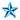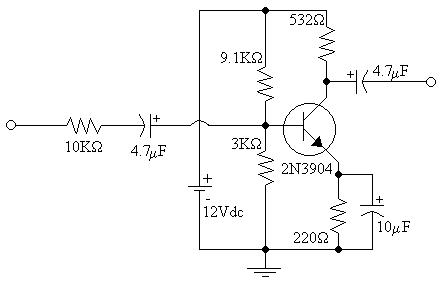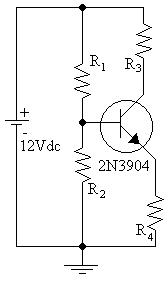EL - 250

Advanced Analog Circuits

Lab #2: Class A Power Amplifier

Andrew Buettner

March 6, 2003Objective

To design and construct a class A single transistor power amplifier. Then to take readings from the amplifier concerning it's operation.Equipment Used

1) Trainer #

2) Oscilloscope #

3) Multimeter #

4) AC function generator #

5) Various components from the EL - 250 lab kitProcedure

A: Circuit Design

1) Assemble the following circuit:2) Calculate the values of R1, R2, R3, and R4 so that the following specifications are satisfied:

i: b = 150

ii: ICQ = 6 - 12ma

iii: VRC = 4.8V

iv: VRE = 1.8V

v: IR1 = IR2 = 20IBQ

B: Circuit Construction and Operation

1) Assemble the following circuit:2) Measure VB, and VC.

3) Apply a 1KHz signal to the input and maximize the input using the oscilloscope as a reference.

4) Measure the voltage at the output, source, and base.

5) Add a 1KW load resistor across the output.

6) Repeat steps 3 and 4.Data

1) Diagram 1: Schematic 1 - Enlarged view2) Diagram 2: Schematic 2 - Enlarged View3) Table 1: Resistor values for circuit biasing

Resistor:

R1

R2

R3

R4

Value:

9.1KW

3KW

220W

532W

4) Table 2: DC Voltages at the Base and collector

Point:

Base

Collector

Emitter

Voltage:

2.84V

6.93V

2.11V

5) Table 3: AC Voltages (No Load)

Point:

Base

Output

Source

Oscilloscope:

.17V

4.8V

1.6V

DMM (RMS):

147.1mV

3.675V

1.023V

6) Table 4: AC Voltage (1KW Load)

Point:

Base

Output

Source

Oscilloscope:

.17V

3.2V

1.6V

DMM (RMS):

147.1mV

2.453V

1.023VAnswers to Lab Questions

1) Q: What is the theoretical values for the gain and efficiency with this circuit?

A: Av = -196; Ai = -.053; Efficiency = 100%

2) Q: What are the actual values of gain and efficiency for this circuit?

A: Av = -28; Ai = -.688; Efficiency = 57.1%

3) Q: Compare the theoretical and actual values.

A:

Theoretical:

Actual:

% Error:

Av:

-196

-28

85.7%

Ai:

-0.053

-0.688

1198%

Efficiency:

100%

57.1%

42.9%Conclusions

From this lab a simple power amplifier can be built to conform to a basic set of operational standards. However, I do not know the source of the exceptionally large amount of error between the theoretical values and the real ones. I did, however, find it interesting that wave form that occurs at the emitter of the transistor. The wave form was identical to that of the base, only slightly smaller.Attachments

1) Original Lab Handout

2) Original Lab Data

3) Calculations